## Search This Blog

### 200 versus 223

We don't know where the notion of applying signal processing algorithms to musical scales originated. Particularly Autocorrelation - which we've seen turn up relatively recently in §6.6 of Walker & Don's "Mathematics and Music: Composition, Perception, and Performance", and in Andrew Duncan's earlier 1991 paper. It is, in any event, intimately related to Allen Forte's Interval Vector (although we will not cover that topic until later).

One wouldn't bother to perform this on a set of distinct integers - our favoured representation of a musical scale - since there's no 'signal' to process there. Every note in the scale is equally available and the distribution of notes would always be uniform.

A set of - possibly non-distinct - intervals, however, re-presents a scale as a spread of harmonies or of 'interesting' melodic transitions. We see this as the basis for David Lewin's insight into the primary importance of the interval - as opposed to the pitch class - within music. It is what distinguishes music (structures built from an alphabet of intervals) from talk (structures built from an alphabet of symbols).

As we know, a scale may be represented as a set of notes - e.g. {B, D, F, A, C, G, E}, where - here - they're presented in an arbitrary order merely to belabour the point that we're talking about a set and not a list) or - more usefully to us with our (now key-independent) 12 tone polygon counting - as a set of integers.

 No ambiguity is introduced by this arbitrary order of integers modulo 12 - it still picks out exactly one (convex) polygon from our clockish representation of any scale.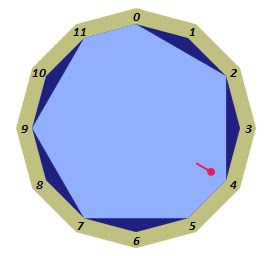← {11, 2, 5, 9, 0, 7, 4} shows {B, D, F, A, C, G, E} as the C Major Scale whereas {2, 5, 8, 0, 3, 10, 7} shows it as A Minor →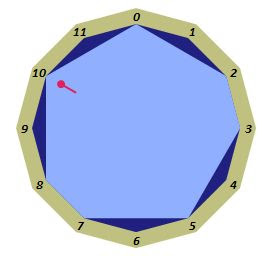However, a scale may also be represented as a set of intervals. For example {2, 1, 2, 2, 2, 1, 2}. The set members are again in no particular order - just two 1s and five 2s. In our dodecatonic universe, the only thing we require of these interval sets is that its members are integers in the range 1 to 12 and that they all sum to 12. The singleton set {12} and the 12 member set {1, 1, 1, 1, 1, 1, 1, 1, 1, 1, 1, 1} are the extreme cases. There are 77 of these sets since there are 77 different ways to get positive integers between 1 and 12 to add up to 12.

Without an ordering, the 7 element set {2, 1, 2, 2, 2, 1, 2} tells us little about the possible polygons that could be constructed, other than that they are heptagons. We can see (in the figure above) that there are indeed two sides of 1 unit and five sides of 'size' 2 (not the actual length of the polygonal side, but the number of vertex leaps). There are many polygons (as always constrained by having one vertex at 0) we could build. We need to introduce an ordering to produce specific polygons.

With the particular intervals we have to hand here, i.e. five 2s and two 1s, it's not too difficult to see that there are only three 'themes', viz 2222211, 2222121, and 2221221 - each of which can be cycled seven times. E.g. the first theme may slide its final 11 backwards six times - 2222112, 2221122, 2211222, 2112222, 1122222, 1222221 - before ending up back at its initial 2222211. Likewise for the remaining themes.

There are, therefore, 21 polygon-scales capable of carrying five whole tone intervals and two semitone intervals. The last of of those listed, i.e. 2221221, represents the seven classical diatonic modes including the Ionian (our modern major scale) and Aeolian (our modern minor scale). As it happens, there are also seven scales - or modes - in use with four consecutive whole tones. These are the Locrian Super (1212222), Javanese (1222212), Locrian Natural (2121222), Melodic Minor (2122221), Hindu (2212122), Lydian Dominant (2221212) and Lydian Augmented (2222121). Of the seven employing five consecutive whole tone intervals, five appear to be in use (common or otherwise) - the Neapolitan Major (1222221), Locrian Major (2211222), Lydian Minor (2221122), another Lydian Augmented (2222112) and Leading Whole Tone (2222211).

Such scale names, by the way, illustrate rather vividly the somewhat unscientific - and certainly haphazard (because history) - terminology in use in music. The Locrian (for example) is almost universally called a 'mode' (and seldom a scale). Whereas both Neapolitan Major and Leading Whole Tone are both accorded the full dignity of scalehood. This is despite the latter's relation to the former being exactly the same as the Locrian is to the Major scale (Ionian mode) - which is to say, one polygon-clicksworth anticlockwise. We're pretty much forced to use the terms 'scale' and 'mode' interchangeably. And the scale (or mode) names are akin to early Linnaean biological species names based on appearance rather than genetics. This is not helpful for taxonomy, nomenclature, or systematics. Which is not to say that more systematic approaches are entirely absent in the more abstruse field of musicology. See, for example, the aforementioned Allen Forte and Elliott Carter for another (sorry about the price).

All of which is a (possibly excessively circuitous) route to the justification for regarding a musical scale (the horizontally-ordered sequence of pitch classes) or chord (the vertically-oriented one), recast as horizontally or vertically oriented sets of intervals, as an object carrying a signal. And, as such, fair game for being subjected to the encharacterising probe of autocorrelation.

You may look up the definition of autocorrelation for yourself, but its essence is as a measure, a spectrum, of the relationships between the notes of the scale and other notes of the scale shifted in sequence. Or - to put it another way - a set of frequencies of all twelve possible intervals (0 to 11) embodied by that scale and the aforementioned Andrew Duncan (loc cit) shows us how to calculate the autocorrelation of any given scale.

Since we're working with polygonal representations of scales - rather than Mr Duncan's beady, necklacy, representations - we demonstrate how to count the frequencies of interval occurrences held inside a scale in our own particular way. Our animation shows the original scale polygon overlaid by a rotating copy (one semitone clockwise each step - or one hour at a time, if you like) to demonstrate the computation for the autocorrelation of the dorian mode of the major scale (12 bit scale #2902). Just count the number of vertices common to the original and its copy at each 'hour'.Thus we may calculate some measure of what we have - hopefully - justified as being a musically relevant property of a scale, as opposed to a merely geometric property of a polygon.

 We can easily fashion this property into a single label by concatenating all twelve values - as the digits 0 to 9 (and, where the necessity - and it's a rare necessity - arises, by using hexadecimal digits A, B and C for 10, 11 and 12) into a single twelve character string - such as 725436263452 for the above.Especially notice the symmetry of the bar chart around the 7th slot (counting the number of 6 semitone intervals).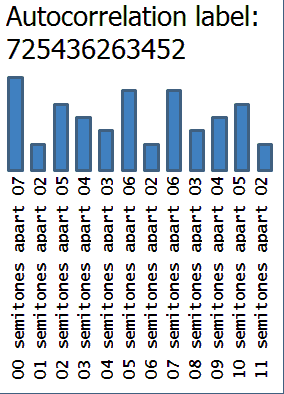## Autocorrelation as Classifier

First, a scale's autocorrelation is independent of its orientation within the 12 hour clock face. The autocorrelation is a property built-in to the polygon, computable solely by rotating a polygon over itself, without reference to which way up it happens to be. It follows from this that there are at least as few autocorrelation labels as there are rotated variants (which musicians may call modes) of any given scale.

### Autocorrelation Merges Rotations (Musical Modes)

Which is to say that 725436263452 labels also the Ionian (aka Major) mode, the Aeolian (aka Minor) mode, as well as the remaining four (Lydian, Locrian, Mixolydian and Phrygian). The seven '2222211' scales are similarly all autocorrelated as 726262626262 and the seven '2222121' as 725444444452. The first 7 is common to all three simply because they are all heptatonic scales. Each have a 2 as the next common value because all 21 scales have two semitone intervals in them. You might expect that - since all scales contain five 2s - the third digits of each of their autocorrelations should, likewise, be 5. But because the 2222211 scales contain two consecutive semitone intervals, there's an extra whole tone leap available in those scales which is unavailable to the other scales where the semitone leaps are separated, never occurring together.

Autocorrelation labeling will thus parallel the classification provided by the '351 equivalencies' because those equivalencies are precisely the rotational ones.

### Autocorrelation Merges Reflections (Musical Inversions)

It also parallels the classification by inversion (the reduction from 351 to 224 obtained by including lateral reflection in the clock's vertical axis). For example, consider a non-symmetric scale such as the non-scalar-invertible Hungarian Major (12 bit scale #2486). Here is its autocorrelation being calculated:

 The autocorrelation label for this heptatonic Hungarian Major scale is - likewise - going to be identical (in this case 733633633633) for its six further (modal) rotational variants.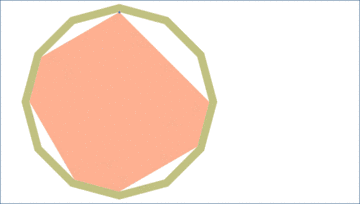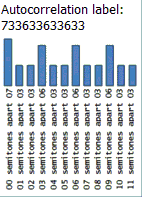Furthermore, performing the same process on the unnamed (so far as we know) scale obtained by reflecting the above Hungarian Major scale in the clock's vertical 0-6 axis results in exactly the same autocorrelation.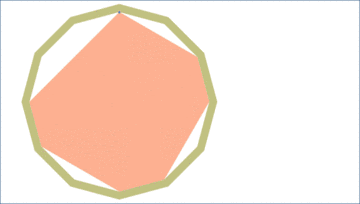Autocorrelations of non-symmetric scales, as well as those of the symmetrical ones, are unaffected by either rotation or reflection. This is because the autocorrelation of any scale which has been reflected in the clock's 0-6 axis is equivalent to rotating anticlockwise during its computation. The counts for the intervals are going to be exactly the same as those done clockwise for its mirror image. This means that autocorrelation calculations applied to all 2048 scales can have no more distinct results than the 223 counted by Reiner's enumeration.

## 200 Scale Equivalences?

Although there can be no more than 223 distinct autocorrelation distributions, however, when we calculate them for all 2048 scales, we find only 200 distinct distributions. This is because 12 pitch classes permit equivalences beyond rotation and reflection. Most of these - 15 of the 23 - occur at the halfway point, with hexatonic scales. Seven pairs of symmetric hexatonic scales, despite being distinctly different polygons, have the same autocorrelation.

## Hexatonic autocorrelates

For example, the two - rather different looking - hexatonic scales below (and of course their further five 'modal' rotations each) have the same autocorrelation, 623234243232. Which is to say that all 12 of these two scales, in their six modes each, share the same interval densities.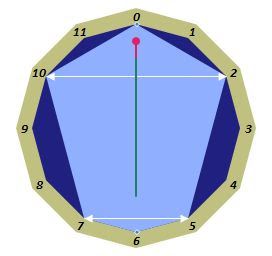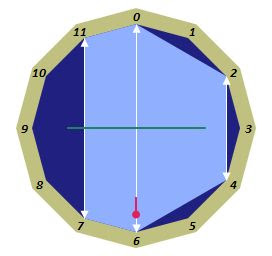Those interval densities are presented in a little more detail below, showing in each case the relevant 'digits' of the autocorrelation 'label' formed from the frequency graph.

semitones
in interval
how many
of them
coming fromlabelled in
autocorrelation
026 scale notes  623234243232
142 minor seconds+2 major sevenths623234243232
263 major seconds+3 minor sevenths623234243232
342 minor thirds+2 sixths623234243232
463 major third+3 minor sixth623234243232
584 perfect fourth+4 perfect fifth623234243232
621 augmented fourth+1 diminished fifth623234243232

Note that the last line of the table is presented as showing an augmented fourth distinct from a diminished fifth, which of course is not the case as both intervals will land on the exact same pitch in the way that they won't when the intervals are, say, major second and minor seventh.

To check on what's being counted, examine one of the rows - say, the '3 major seconds + 3 minor sevenths' one. In the following descriptions we will always travel clockwise.

In the left hand scale we can find the three major seconds from 0 to 2, from 5 to 7 (hurdling the 6) and from 10 to 12 (i.e. 0). The minor sevenths are, correspondingly, from 0 to 10 (the long way round), from 7 to 5 (again the long way round) and finally from 2 to 12 (i.e. 0). In the right hand scale, the three major seconds are from 0 to 2, from 2 to 4 and from 4 to 6. And the minor sevenths are from 2 to 0, from 4 to 2 and from 6 to 4 - all three being taken the long way round the clock. After all that, we equate the minor seventh interval to the major second since the pitch classes at each end of the interval are 2 semitones apart. E.g. in the left scale from 0 to 10 the quick way round, anticlockwise.

All inverted asymmetric scale pairs, we already know, automatically share the same autocorrelation. But there are eight further asymmetric hexatonic scales (with their reflections) which share the same autocorrelations as eight others. Thus these eight particular autocorrelation distributions each cover 24 rather than 12 distinct scales.

## Heptatonic autocorrelatives (and Pentatonic Duals)

Autocorrelative equivalences don't end with hexatonic scales. Two distinct heptatonic scales (with interval patterns of 1141212 and 1113132, each of which have their septet of modal rotations) with differently shaped heptagonal representations share the same autocorrelation - of 743444444434 - as well as with their mirror scales (with reflected interval patterns of 2121411 and 2313111). As far as we know, none of these 28 modes appear to be in use in the world.

1141212111313221214112313111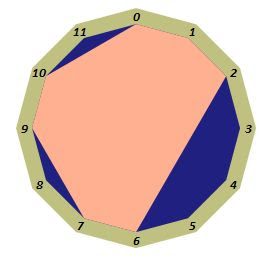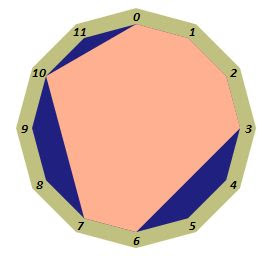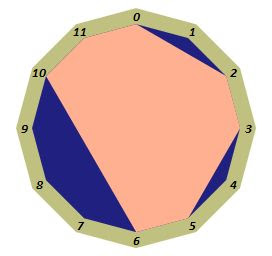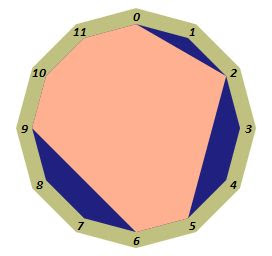And, as numbers of scales of fewer than 6 notes symmetrically follow those of more than 6, there is a corresponding quartet of pentatonic scales (with interval patterns 33411, 25131, 11433 and 13152) where their 20 modal variants all share an autocorrelation of 521222222212. As before, none of these scales appear to be in use.

33411251311143313152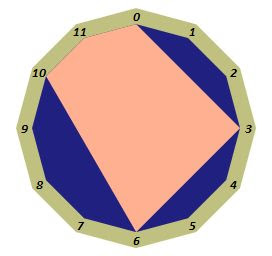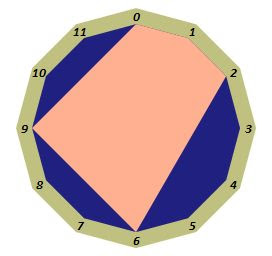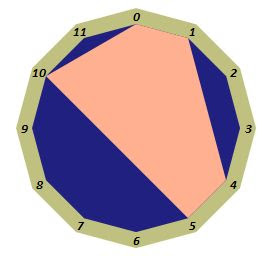It will perhaps come as no great surprise to note that the pentatonic and heptatonic scales above are 'antiscales' of each other in that the five unused vertices of the heptagon match the five vertices of the pentagon below it (appropriately rotated so that 12 o'clock is, as always, occupied by a vertex).

In contrast to the hexatonic case, where 7 distinct autocorrelations served 7 pairs of different symmetric scales, there is only 1 pair of symmetric heptatonic scales (with interval patterns 3112113 and 1214121) sharing the same autocorrelation of 743454245434. With all modes included, that's 14 heptagons covered by one characterising value. And the single (symmetrically expected) pair of pentatonic (anti)scales sharing an autocorrelation of 521232023212 have interval patterns of 41214 and 13431.

Mixing things up a bit, a single, rather special heptatonic autocorrelation value, 744434443444, covers three heptagons. It links the symmetric scale modes with interval pattern 1132311 and its rotations, and the non-symmetric scale modes with interval pattern 1114212 and its rotations, and its mirror image 2124111. There is, of course, a pentatonic 'dual' for each of these and - as you can probably guess - they are the 'antiscales' to these three heptatonic patterns. The autocorrelation is 522212221222 and the interval patterns are 21612 (symmetric pentagon) 11532 (non-symmetric) and 23511 (its mirror) - although given that the patterns of the heptagons also tell you which vertices are not included, you should be able to infer the pentatonic interval patterns from the gaps in the heptatonic ones (images below).

## An Octatonic autocorrelate (and its tetratonic dual)

By the time we get up to 8 note scales (and, correspondingly, down to 4) there are too few possibilities to support very many autocorrelation distributions and - in fact - we reach one case only. The distribution is 855555655555 and - intervalwise - it's as evenly spread as it could possibly get. It covers four octagons, in each of their 8 rotations, and these are a pair of non-symmetric polygons and their mirror images, The interval patterns are 11321121 (and its mirror 12112311) and 13122111 (and its mirror 11122131). The second pattern, with its four consecutive semitones, looks pretty uninspiring but - being octatonic - there's slightly more room for longer runs of semitones. And this scale is actually in use in the world as the Bebop Dominant Flatnine. One might suppose, therefore, that the differently shaped but identically autocorrelated scale with interval pattern 12112311 might serve as a substitute. As always, there's a match for these scales in the tetratonic (anti)scales - two pairs of non-symmetric tetramodals. We leave it as an exercise for the student to find them from the vertex gaps of their four dual octatonics.

11321121121123111312211111122131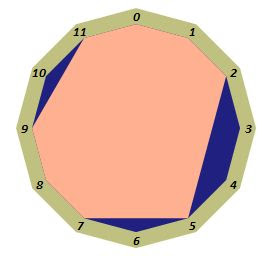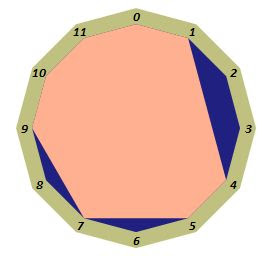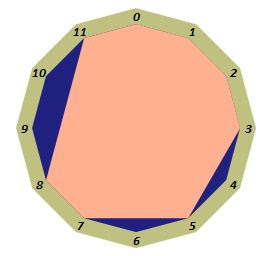bebop dominant flatnine and its 'monoclonally intervalled' siblings

We can add this information into the enumeration table, showing a reduction of the 223 transpose/inverse equivalences to the 200 autocorrelation (scale interval distribution) equivalences. The hypothesis is that, given a particular interval spread from an elected autocorrelation, randomly generated sequences of notes and chords built from any pair of identically autocorrelated scales (in the same tonic) should - in some way - sound indistinguishable in sufficiently long exercises. It would be interesting to design an experiment to test this hypothesis.

Number of notes in scale
123456789101112total
11155165330462 46233016555111 2048
1619436680 664319611 351
symmetric:=
165151020 10155611 95
non-symmetric pairs: (i.e. to be doubled in the total)+
007142830 28147000 256
95 invertible + 128 noninvertible mirror-equivalent scales
1612293850 382912611 223
Identically Autocorrelated Scales
1612283635 362812611 200
distinct autocorrelations for invertible scales=
16515913 9155611 86
distinct autocorrelations for noninvertible scales+
007132722 27137000 116
distinct autocorrelations for both invertible and noninvertible scales-
000010 100000 2

The final row picks out the two special autocorrelations mentioned earlier (labelled 744434443444 and 522212221222) which attach to the symmetric heptatonic scale #3988 with non-symmetric scale pair #3944 and #3862 (and all their further 6 rotations each) and the symmetric pentatonic scale #3424 with non-symmetric scale pair #3728 and #3602 (and all their further 4 rotations each).

Heptatonic scales with identical autocorrelation 744434443444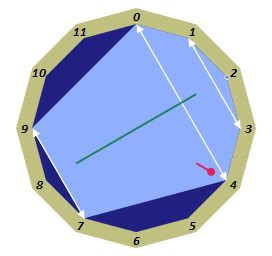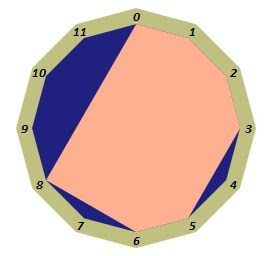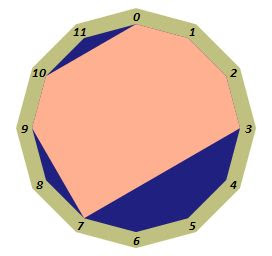Pentatonic scales with identical autocorrelation 522212221222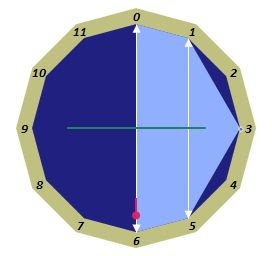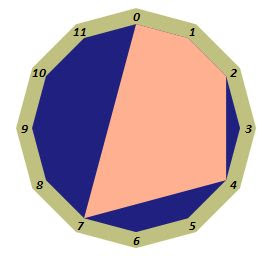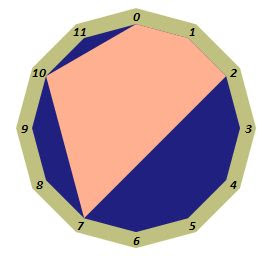Note that the 'identifiers' in use before the introduction of autocorrelation have had reconstructive utility. For example, the 'which notes are in the scale' identifier is the 12 digit bitstring (with the first digit - representing the tonic note of the scale - always 'on') tells you immediately how to draw the polygon starting from 12 o'clock. Likewise the decimal numbers 2048 to 4095 can be used to draw a polygon working anticlockwise from 11 o'clock (if it's odd, 11 o'clock is in else it's out, then divide by two - forgetting about any halves - as you move to ten o'clock. If this new number is odd, then 10 o'clock is in else it's out .. repeat until zero o'clock). And the 'interval pattern' which, again applied clockwisely from 12 o'clock, tells you how to draw a polygon by the length of the 'hour jump' between vertices.

Not so the autocorrelation, however. The 12 character label (or the 12 counts of unisons, minor seconds, seconds, ... major sevenths available) isn't of much utility in uniquely recovering a polygon representing its distribution of intervals, especially for scales containing between 4 and 8 pitch classes (arguably the only interesting ones).

Having said that, one might imagine that all of this has been an awful lot of trouble to go to only to find that certain dissimilar looking polygons, representing those aforementioned scales built from 4 to 8 notes, share some distinct interval-based property. But there is, after all, something musical buried in these properties and - when the autocorrelation is recycled - we can actually tell 'good' scales from 'bad' ones, we can order them to find the 'best' octatonic scale, for example, or the 'worst' hexatonic one. Naturally these are somewhat ridiculous value judgements in the context of music, but the metrics available from recycled autocorrelations are nevertheless real, and usable.

In case you're interested, the worst possible hexatonic scale is in fact that well known staple of French Impressionism, carrying some of the prettiest music around, the whole-tone scale.

Now that we've mentioned 'harmoniousness' on this reduction from 2048 down to 200, we can pause for a while at this plateau. We may consider reordering this enumeration with that harmoniousness in mind. It's a risky pause for the serious minded - all we've done so far has been about counting congruent or similar polygons. Where's the actual music?